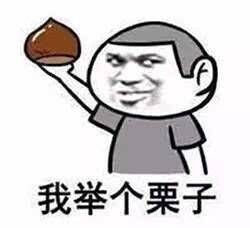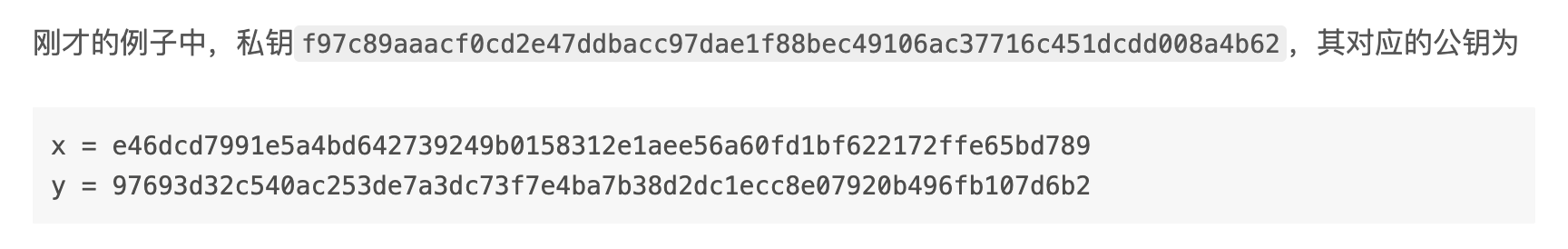$$y^2 \equiv x^3 + ax + b \pmod{p}$$

• 已知曲线上的点 $P = (x_P, y_P)$ 和 $Q = (x_Q, y_Q)$，求点 $R = P + Q$
• 已知整数 $k$，求点 $K = k \cdot G$

# 点的（代数）加法

$$x = (m^2 - x_P - x_Q) \bmod{p}$$

$$y = (y_P + m(x_R - x_P)) \bmod{p} = (y_Q + m(x_R - x_Q)) \bmod{p}$$

$$m = (y_P - y_Q)(x_P - x_Q)^{-1} \bmod{p}$$

$$m = (3 x_P^2 + a)(2 y_P)^{-1} \bmod{p}$$

# 标量积（数乘运算）

$$k \cdot P = \underbrace{P + P + … + P}_{k\ 个}$$$$141_{10} = 10001101_{2}$$

$$141P = 2^7P + 2^3P + 2^2P + 2^0P$$

• 计算 $2P = P + P$，即 $2^1P$
• 计算 $4P = 2P + 2P$，即 $2^2P$
• 计算 $8P = 4P + 4P$，即 $2^3P$
• ……
• 计算 $2^7P$
• 计算 $2^7P + 2^3P + 2^2P + 2^0P$ 得到 $141P$

# 验证# 完整代码

ec_point_operation.py